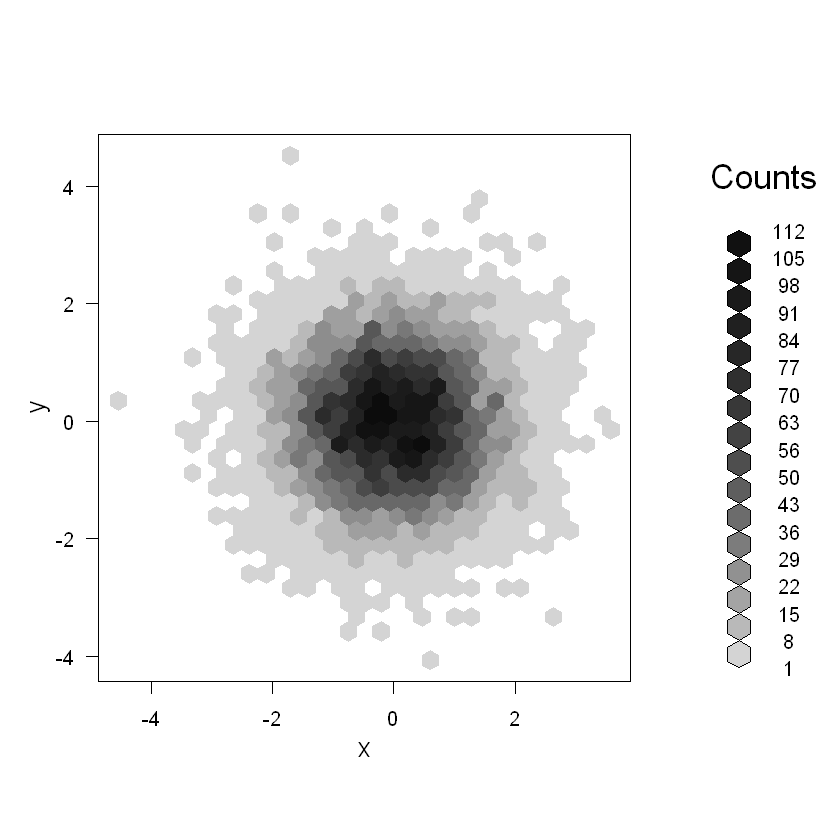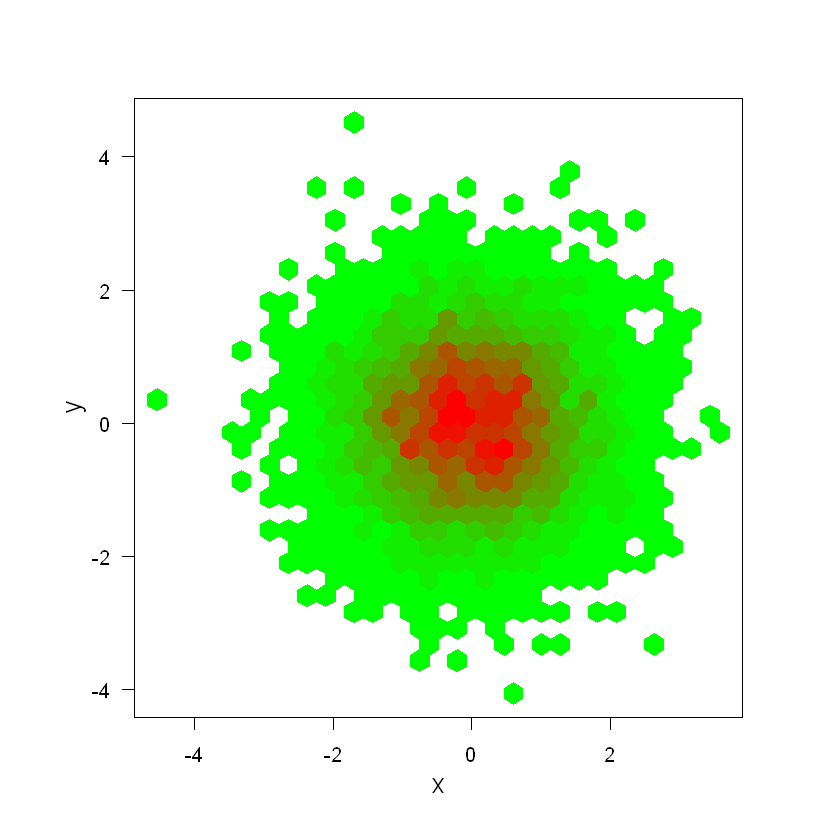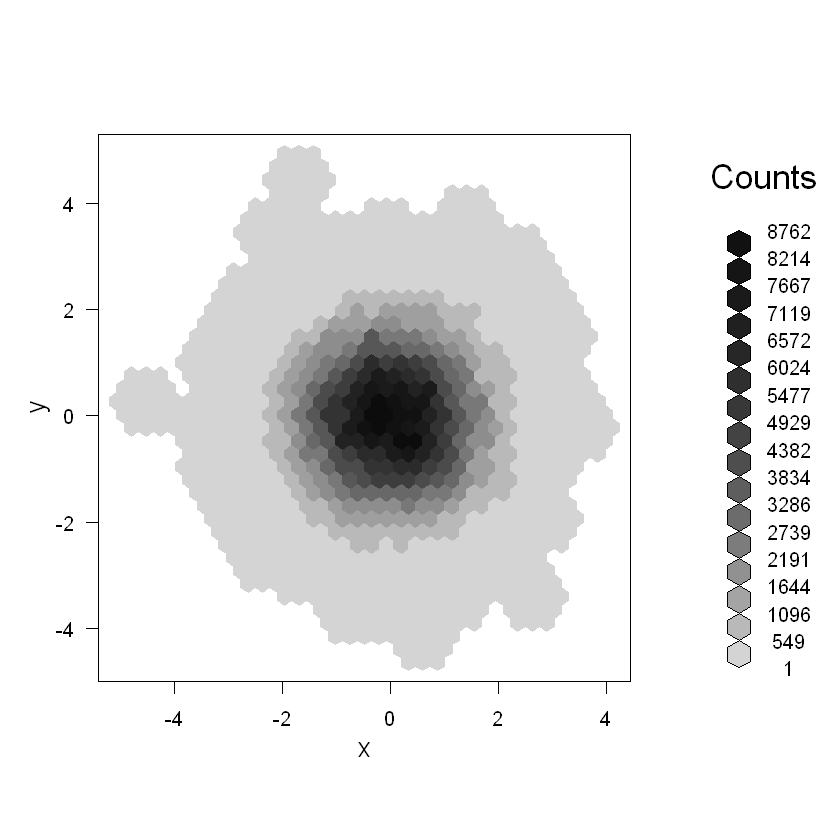GeeksforGeeks App
Open AppBrowser
Continue

# HexBin Plot using hexbin Packages in R

In this article, we are going to see how to plot HexBin Plot using R Programming Language.

HexBin plot is also known as hexagonal bin plot and it looks like a honeycomb with different square shading. And each square shading notes with different data points graphed in two dimensions coordinated and square represents a collection of points.

To plot HexBin Plot we will use hexbin Packages. It is used to Binning and plot functions for hexagonal bins. To install this package use the following commands:

`install.packages("hexbin")`

Note: The object in hexbin cannot be coerced to type ‘double’

Syntax:

hexbin(x, y)

Where, x, y vectors giving the coordinates of the bivariate data points to be binned. Alternatively a single plotting structure can be specified: see xy.coords. NA’s are allowed and silently omitted.

Here we will plot a basic hexagonal bin plot using hexbin package. For this, we will create a double type dataset and then pass as an argument into hexbin() methods and then plot it using plot() methods.

Example: Basic hexbin plot

## R

 `library``(hexbin)` `set.seed``(153)` `x <- ``rnorm``(10000)``y <- ``rnorm``(10000)` `bin <- ``hexbin``(x,y)` `plot``(bin)`

Output:## Color customization:

Basically, there are not any built-in methods or attributes in this package for color customization. We will use colorRampPalette() methods color range between two colors specified points

Syntax:

colorRampPalette(color)

Example: Color customization

## R

 `library``(hexbin)` `# for color palette``library``(RColorBrewer)``set.seed``(153)` `x <- ``rnorm``(10000)``y <- ``rnorm``(10000)` `bin <- ``hexbin``(x,y)` `plot``(bin, main=``""` `, colramp=``colorRampPalette``(``c``(``"Green"``, ``"red"``)) ,``     ``legend=F )`

Output:## Smooth hexagonal

smoother uses the immediate center cell, immediate neighbors, and second neighbors to smooth the counts.

Example: Smooth hexagonal

## R

 `library``(hexbin)` `set.seed``(153)` `x <- ``rnorm``(10000)``y <- ``rnorm``(10000)` `bin <- ``hexbin``(x,y)` `smbin <- ``smooth.hexbin``(bin)``plot``(smbin)`

Output:My Personal Notes arrow_drop_up